# Polynomial NCERT Exercise 2.3

## NCERT Solutions for Class 10 Maths Chapter 2 Exercise 2.3

In this page we have NCERT Solutions for Class 10 Maths Chapter 2 Polynomial for Ex 2.3 on page 36. Hope you like them and do not forget to like , social_share and comment at the end of the page.
Question 1
Divide the polynomial p(x) by the polynomial g(x) and find the quotient and remainder in each of the following:
(i) p(x) = x3 – 3x2 + 5x – 3, g(x) = x2 – 2
(ii) p(x) = x4 – 3x2 + 4x + 5, g(x) = x2 + 1 – x
(iii) p(x) = x4 – 5x + 6, g(x) = 2 – x2
(i) p(x) = x3 – 3x2 + 5x – 3, g(x) = x2 – 2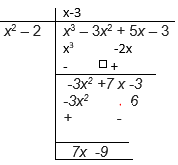Quotient = x-3 and remainder 7x – 9

(ii) p(x) = x4 – 3x2 + 4x + 5, g(x) = x2 + 1 – x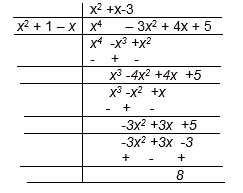Quotient = x2 + - 3 and remainder 8

(iii) p(x) = x4 – 5x + 6, g(x) = 2 – x2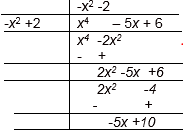Quotient = -x2 -2 and remainder -5x +10

Question 2.
Check whether the first polynomial is a factor of the second polynomial by dividing the second polynomial by the first polynomial:
(i) t2 – 3,  2t4 + 3t3 – 2t2 – 9t – 12
(ii) x2 + 3x + 1, 3x4 + 5x3 – 7x2 + 2x + 2
(iii) x3 – 3x + 1, x5 – 4x3 + x2 + 3x + 1
(i) t2 – 3, 2t4 + 3t3 – 2t2 – 9t – 12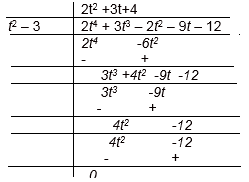So
t2 – 3 exactly divides 2t4 + 3t3 – 2t2 – 9t – 12 leaving no remainder. Hence, it is a factor of
2t4 + 3t3 – 2t2 – 9t – 12.

(ii) x2 + 3x + 1, 3x4 + 5x3 – 7x2 + 2x + 2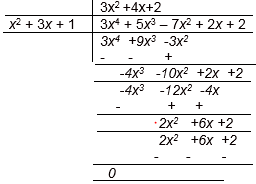x2 + 3x + 1 exactly divides 3x4 + 5x3 – 7x2 + 2x + 2 leaving no remainder. Hence, it is factor of 3x4 + 5x3 – 7x2 + 2x + 2.

(iii) x3 – 3x + 1, x5 – 4x3 + x2 + 3x + 1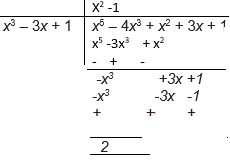x3 – 3x + 1 didn't divides exactly x5 – 4x3 + x2 + 3x + 1 and leaves 2 as remainder. Hence, it not a factor of x5 – 4x3 + x2 + 3x + 1.

Question 3.
Obtain all other zeroes of 3x4 + 6x3 – 2x2 – 10x – 5, if two of its zeroes are √(5/3) and - √(5/3).
p(x) = 3x4 + 6x3 – 2x2 – 10x – 5
Since the two zeroes are √(5/3) and - √(5/3).
Therefore
[x-√(5/3)]  and  [x+√(5/3)]   are factors of the polynomial p(x)
So [x-√(5/3)]  [x+√(5/3)]   =(x2 -5/3)  is a factor of the polynomial p(x)
Let’s divide the p(x)  by (x2 -5/3)   to get remaining factors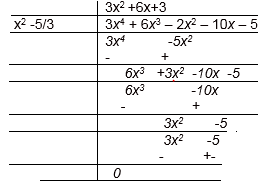So
P(x)= 3x4 + 6x3 – 2x2 – 10x – 5
=(3x2 +6x+3)(x2 -5/3)
=3(x2 +2x+1)( x2 -5/3)
We factorize x2 + 2+ 1
= (+ 1)2
Therefore, its zero is given by x + 1 = 0
x = -1
So the other  2 zeroes are x = - 1.
Hence, the zeroes of the given polynomial are √(5/3) and - √(5/3), - 1 and - 1.

Question 4.
On dividing x3 - 3x2 + x + 2 by a polynomial g(x), the quotient and remainder were x - 2 and
-2x + 4, respectively. Find g(x).
Here in the given question,
Dividend = x3 - 3x2 + x + 2
Quotient = x - 2
Remainder = -2x + 4
Divisor = g(x)
We know that,
Dividend = Quotient × Divisor + Remainder
⇒ x3 - 3x2 + x + 2 = (x - 2) × g(x) + (-2x + 4)
x3 - 3x2 + x + 2 - (-2x + 4) = (x - 2) × g(x)
x3 - 3x2 + 3x - 2 = (x - 2) × g(x)
g(x) =  (x3 - 3x2 + 3x - 2)/ (x - 2)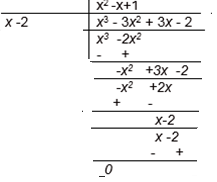g(x) = (x2 - x + 1)

Question 5
Give examples of polynomial p(x), g(x), q(x) and r(x), which satisfy the division algorithm and
(i) degree p(x) = degree q(x)
(ii) degree q(x) = degree r(x)
(iii) degree r(x) = 0
According to the division algorithm, if p(x) and g(x) are two polynomials
with g(x) ≠ 0, then we can find polynomials q(x) and r(x) such that
p(x) = g(x) × q(x) + r(x),   --(A)
where r(x) = 0 or degree of r(x) < degree of g(x)
(i)
degree p(x) = degree q(x)
From equation (A), then r(x)=0 and q(x)  =constant term
Here Let us assume the division of 9x2 + 6x + 3 by 3
Here, p(x) = 9x2 + 6x + 3
g(x) = 3
q(x) = 3x2 +2x + 1
r(x) = 0
Degree of p(x) and q(x) is same i.e. 2.
Checking for division algorithm,
p(x) = g(x) × q(x) + r(x)
Or, 9x2 + 6x + 3= 3 × (3x2 +2 + 1)
Hence, division algorithm is satisfied.

(ii)
Now degree q(x) = degree r(x)
Let us assume the division of x3x by x2,
Here, p(x) = x3 + x
g(x) = x2
q(x) = x and r(x) = x
Clearly, the degree of q(x) and r(x) is the same i.e., 1.
Checking for division algorithm,
p(x) = g(x) × q(x) + r(x)
x3 + x = (x2 ) × x + x
x3 + x = x3 + x
Thus, the division algorithm is satisfied.
(iii)
degree r(x) = 0
Let us assume the division of x3+ 5 by x2.
Here, p(x) = x3 + 5
g(x) = x2
q(x) = x and r(x) = 5
Clearly, the degree of r(x) is 0.
Checking for division algorithm,
p(x) = g(x) × q(x) + r(x)
x3 + 5 = (x2 ) × + 5
x3 + 5 = x3 + 5
Thus, the division algorithm is satisfied

## Summary

1. NCERT book Solutions for Class 10th Maths:Polynomials Exercise 2.3 has been prepared by Expert with utmost care. If you find any mistake.Please do provide feedback on mail. You can download the solutions as PDF in the below Link also
2. This chapter 2 has total 4 Exercise 2.1 ,2.2,2.3 and 2.4. This is the Third exercise in the chapter.You can explore previous exercise of this chapter by clicking the link belowGo back to Class 10 Main Page using below links

### Practice Question

Question 1 What is $1 - \sqrt {3}$ ?
A) Non terminating repeating
B) Non terminating non repeating
C) Terminating
D) None of the above
Question 2 The volume of the largest right circular cone that can be cut out from a cube of edge 4.2 cm is?
A) 19.4 cm3
B) 12 cm3
C) 78.6 cm3
D) 58.2 cm3
Question 3 The sum of the first three terms of an AP is 33. If the product of the first and the third term exceeds the second term by 29, the AP is ?
A) 2 ,21,11
B) 1,10,19
C) -1 ,8,17
D) 2 ,11,20